# How to summarize a column

## Description

When provided with a dataset in which you want to focus on one column, how would you compute descriptive statistics for that column?

## Solution, in Excel

View this solution alone.

Let’s assume you have some data in a single column of an Excel workbook. We show the first 10 rows (out of 27) for some example data below, but we assume you are applying what we cover here to your own real data.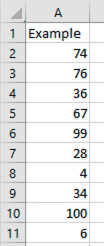To compute descriptive statistics, you will need the Data Analysis Toolpak. If you’ve never enabled it before, see these instructions from Microsoft on how to do so.

On the Data tab, click the Data Analysis button, shown below.From the list of tools it provides, choose Descriptive Statistics, as shown below, then click OK.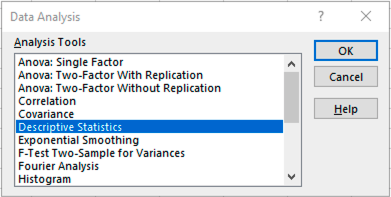Highlight all the cells in your column as input (excluding the column header, if any), then check the “Summary statistics” checkbox, as shown below. Then click OK.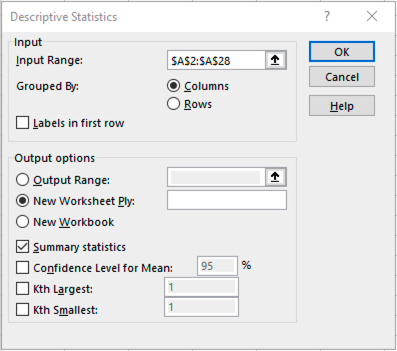Excel will create a new sheet that reports your column’s mean, median, variance, and more, as shown below.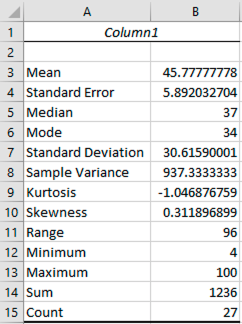To get a report of the unique values in your column and the frequency of each, you can use a pivot table. Highlight your column of data, then on the Insert tab, choose Pivot Table, as shown below.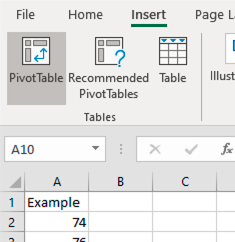Drag your column’s name from the list of pivot table fields down into both the Rows and Values areas, as shown below.From the drop-down under Values, choose “Value field settings…” and change Sum to Count, as shown below. Then click OK.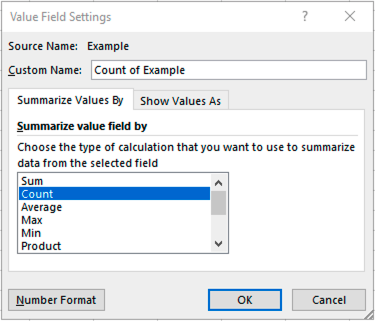Your pivot table will now contain the desired report. The first few rows with our example data look like the following. Most data points appear only once, but 6 appears twice and 34 appears three times.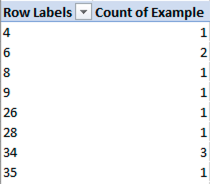See a problem? Tell us or edit the source.

## Solution, in Python

View this solution alone.

The solution below uses an example dataset about the teeth of 10 guinea pigs at three Vitamin C dosage levels (in mg) with two delivery methods (orange juice vs. ascorbic acid). (See how to quickly load some sample data.)

1
2
from rdatasets import data
df = data('ToothGrowth')


Let us consider qualitative and quantitative variables separately.

Consider the qualitative column “supp” in the dataset (which type of supplement the animal received). To count the distribution of each categorical value, use value_counts():

1
2
df['supp'].value_counts()
# Or use df['supp'].value_counts(normalize = True) for proportions instead.

1
2
3
4
supp
VC    30
OJ    30
Name: count, dtype: int64


The output says that there are 30 observations under each of the two levels, Orange Juice and Ascorbic Acid.

If you wish to jointly summarize two categorical columns, provide both to value_counts():

1
df[['supp','dose']].value_counts()

1
2
3
4
5
6
7
8
supp  dose
OJ    0.5     10
1.0     10
2.0     10
VC    0.5     10
1.0     10
2.0     10
Name: count, dtype: int64


This informs us that there are 10 observations for each of the combinations.

Now consider the quantitative column len in the dataset (the length of the animal’s tooth). We can compute summary statistics for it just as we can for a whole dataframe (as we cover in how to compute summary statistics).

1
df['len'].describe() # Summary statistics

1
2
3
4
5
6
7
8
9
count    60.000000
mean     18.813333
std       7.649315
min       4.200000
25%      13.075000
50%      19.250000
75%      25.275000
max      33.900000
Name: len, dtype: float64


The individual functions for mean, standard deviation, etc. covered under “how to compute summary statistics” apply to individual columns as well. For example, we can compute quantiles:

1
df['len'].quantile([0.25,0.5,0.75])   # These chosen values give quartiles.

1
2
3
4
0.25    13.075
0.50    19.250
0.75    25.275
Name: len, dtype: float64


See a problem? Tell us or edit the source.

## Solution, in R

View this solution alone.

The solution below uses an example dataset about the teeth of 10 guinea pigs at three Vitamin C dosage levels (in mg) with two delivery methods (orange juice vs. ascorbic acid). (See how to quickly load some sample data.)

1
df <- ToothGrowth


Let us consider qualitative and quantitative variables separately.

Consider the qualitative column “supp” in the dataset (which type of supplement the animal received). To count the distribution of each categorical value, use table():

1
table(df$supp) # OR summary(df$supp)

1
2
OJ VC
30 30


The output says that there are 30 observations under each of the two levels, Orange Juice and Ascorbic Acid.

If you wish to jointly summarize two categorical columns, provide both to table():

1
table(df$supp, df$dose)

1
2
3
0.5  1  2
OJ  10 10 10
VC  10 10 10


This informs us that there are 10 observations for each of the combinations.

Note: If there are more than 2 categorical variables of interest, you can use ftable() instead.

Now consider the quantitative column len in the dataset (the length of the animal’s tooth). We can compute summary statistics for it just as we can for a whole dataframe (as we cover in how to compute summary statistics).

1
summary(df$len)  1 2 Min. 1st Qu. Median Mean 3rd Qu. Max. 4.20 13.07 19.25 18.81 25.27 33.90  The individual functions for mean, standard deviation, etc. covered under “how to compute summary statistics” apply to individual columns as well. For example, we can compute quantiles: 1 quantile(df$len) # quantiles

1
2
0%    25%    50%    75%   100%
4.200 13.075 19.250 25.275 33.900